# Overview of setting up Implied Volatility Surfaces in Endur/Findur

There are a number of ways of setting up Volatility Surfaces (as opposed to just ATM Vols). This Article provides an overview and concentrates specifically on Delta defined surfaces.

## Delta Dimension: Standard

When Delta is used as a volatility lookup parameter (2nd dimension, the 1st being the expiry), a circular dependency is introduced, as the option’s Delta is a function of the volatility and the volatility used by the option is a function of the option’s Delta.

Below figure shows a Call Delta based surface (whether its Call or Put based values is a setting on the Volatility Definition for the delta dimension) and also the Put/Call Parity relationship (more of which later in this Article).(Fig1)

Endur has two methods of resolving this.

### Method 1: Equivalent Strike lookup method

The Strike Ratio of the Deal (Value 1) is matched off against the interpolated Equivalent Strike Ratio calculated on the Vol Surface (Value 2). The match off steps are as follows:

• Value 1 : Strike Ratio Calculated from Deal values : Calculates the Option Strike Ratio of the deal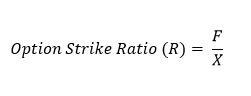(Fig2)

Where F is the Price and X is the Strike from the deal.

• Value 2 : Equivalent Strike Ratio Calculated on the Vol Surface : Calculates the Equivalent Strike Ratio on the whole surface of the Vol Curve. It is first calculated at each Gridpoint and then Interpolated between the Gridpoints dependent on the interpolation method selected. When setting up the Equivalent Strike Ratio method on the Delta Dimension the Gridpoint Usage (Call / Put) and Grid Point Form (Forward / Future) determines the Equivalent Strike Ratio calculation on the Vol Surface. Below we assume it’s set as a Call Future. Endur uses the Put / Call Parity ( more of this later ) to be able to match off a Call or Puts Option Strike Ratio ( R ) against the Equivalent Strike Ratio on the vol surface.(Fig3)

This is calculated at each Volatility Gridpoint level using

• r ( delta ) from the Column Definition
•as the Volatility Input

• T is the time to expiry from the Volatility Gridpoint (row) Date, not the time to expiry of the Deal. This is a significant detail which will influence how the volatility gridpoints on the Time dimension are setup ( more on this later )

You can see this calculated value from the Volatility Input screen by going to View à Equivalent Strike Ratio.

• Then Value 1 is matched off against the interpolated Value 2 on the Vol Surface. i.e Endur looks up the deals Option Strike Ratio on the interpolation of the Call Equivalent Strike Ratio and picks the Vol at that interpolated point.

Note that whilst this method is efficient, OpenLink recommend that the boundaries of the Delta dimension are wide as one of the observed deficiencies of the strike-ratio based method is for extrapolated values beyond the input boundaries.

### Method 2: Iterative Lookup method

The basic concept for the iterative method is that we want to find the point on the volatility surface where the option’s Delta for a given volatility corresponds to the Delta on the Delta axis of the volatility surface. Given that we need to know the option’s volatility value to compute its Delta, we do not know the Delta in advance of the volatility lookup. Therefore this Iterative method allows us to find the volatility for a given Delta that produces that same Delta value for the option.

Endur uses Put/Call Parity (more on this later below) so we will use the Call setting as an example.

The Iterative Method uses the closed form solution to calculate the Delta of the deal :Where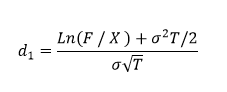(Fig4 )

• F , X are from the deal
•will be the itterative volatility input in the loop described below

• T is the time to expiry from the Volatility Gridpoint (row) Date, not the time to expiry of the Deal. This is a significant detail which will influence how the volatility gridpoints on the Time dimension are setup ( more on this later )

Given the above definitions, the following describes the Iterative Method

• Step 1: A seed Input Delta is selectedfrom the Volatility surface, and then its aasociated seed interpolated volatilityis looked up ( depending on whatever interpolation method has been selected ).(Fig5)

• Step 2: This volatility value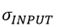( associuated with) is input into the equation above ( Fig 4 ) to calculate the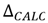.

• Step 3:andare then compared with the difference between them being an error term. This error term is used in the numerical solving routine.

• Step 4: Another next guessis selected and the process repeated untill the error term ( the difference between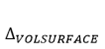and) converges to a specified tolerance. The logic for choosing the next guess is a function of the numerical solving routine used ( which in Endur is the Newton-Raphson method).

Pro: According to Openlink this method appears to address one of the observed deficiencies of the strike-ratio based method for extrapolated values beyond the input boundaries.

Con: Computationally intensive

## Delta Dimension: RR approach

A delta dimension doesn’t have to be defined as simple delta points (above). In some markets it’s common to quote the volatility skew / curvature in terms of Risk Reversal and Delta Strangle.

The names comes from option strategies for RR and DS. This still falls under the Delta dimension in Endur (and still has the Equivalent Strike Ratio and Iterative Method as its two lookup methods) , with the Grid Point Usage field set to “Risk rev & Butterflies”.

Both these values are a way to measure the shape of the Volatility Smile.

Risk Reversal gives a measure of Volatility Skew. At the 25 Delta points:

• 25 Delta Risk Reversal = Vol 25 Delta Call – Vol 25 Delta Put

Delta Strangle gives a measure of the curvature of the volatility smile. At the 25 Delta points:

• 25 Delta Strangle = ( Vol 25 Delta Put + Vol 25 Delta Call ) / 2 – Vol ATM(Fig6)

## Strike Dimension

As an alternative to setting up the 2nd Dimension as a Delta dimension, we can set up a Strike dimension.

There are 4 options available under this Strike Dimension type: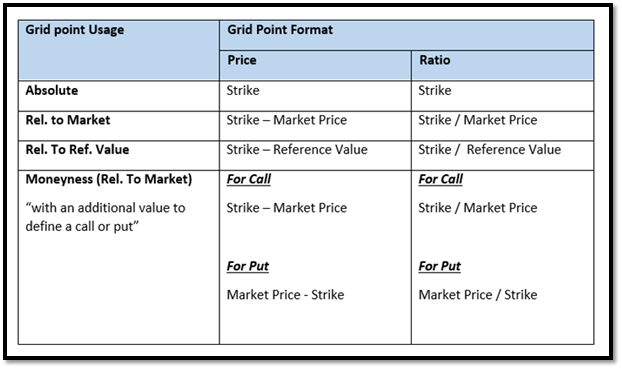(Fig7)

(The Reference Value seems to be constrained to a Vol definition level constant)

Pro: Is much simpler to reconcile where the option Volatility has been picked up from. Also there is less of an issue around matching the Time dimension gridpoints ( T ) to the Option Expiry Date ( see later in this article ).

Con: Doesn’t match how the markets / FO quote Volatilities. In addition some of the choices above means that Strike dimension columns would constantly need adding / deleting / amending / adjusting as the underlying market prices and strikes change.

## Delta Surface Put / Call Parity

When Delta dimension is selected, the Volatility Surface is defined as either Call or Put. The reason why the Vol Surface only needs to be set up for one or the other, is because of the Put / Call parity.(Fig8)

Endur utilises this to ensure that the Volatility lookup for a Call and a Put (with the same Strike on same Underlying and Time to expiry) is exactly the same.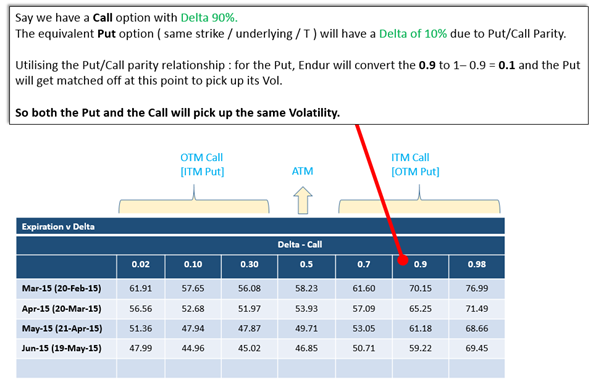(Fig9)

## FO Trader v Endur view of Volatility Surface

There is a potential usability issue with the way in Endur the Delta Vol Surface has to be defined either as all Call or as all Put. That’s because some markets / traders are used to seeing the Vol Surface displayed as OTM Calls and OTM Puts (as these are the most liquid).(Fig10)

Essentially it’s the same Vol Surface – but the traders would view this as flipped (left to right and vice versa) compared to how it’s viewed in Endur.

## Delta Surface Issues

### Expiration Dimension Issue

With a Delta Surface defined, both the Iterative and Equivalent Strike Ratio methods require the Volatility Gridpoint Dates on the Expiration dimension to be the underling Option Expiry date sequence for accurate Volatility lookups.

This stems from the value for “T” (time to expiry) used in the calculations in Fig 3 (Volatility Surface Equivalent Strike Ratio calc.) and Fig 4 (Iterative Option Delta calc.). This value is picked up from the Volatility Gridpoint Date, rather than the Option Expiry Date. What this means with both methods, is that the Volatility Gridpoint Dates need to match the Option Expiry Date to create an accurate lookup.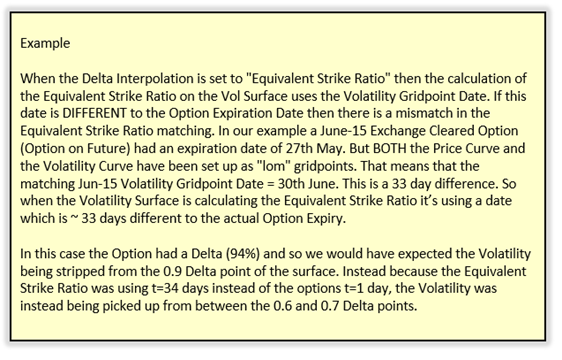So what this means is that the Volatility Gridpoint Dates need to be based on the Option Expiry Date sequence.

#### Exchange Options on Futures

There are two different models on how Endur clients set up the Price curves for the underlying Futures. The underlying Future curve can either be set up as the Future expiry date sequence, or “lom”. The latter seems to be part of the ‘standard’ OpenLink Endur package for European market Futures.

In both cases for the Expiration Dimension:

• the Volatility Gridpoint Dates need to be based on the Option Expiry Date sequence ( it’s perfectly possible to have the Price and Vol curves using different date sequences as the vol lookup on the date axis can be controlled – see bullet point immediately below )

• the Gridpoint Usage needs to be “Option Expiration Date” ( this ensures that the options deals expiration date is used to match against the Volatility Gridpoint Date to get the correct row match )

For Options on Futures you could potentially live with the typical 2 day difference between the Future Expiry Date and Option Expiry Date and use the former.

#### OTC Options

The observation above that the Volatility Gridpoint Dates need to be based on the Option Expiry Date sequence still holds, but it’s more difficult to ensure this for OTC options as there may not be a standard option exercise date logic.

It may well be that a common expiry date logic exist – in which case this should be used.

But additional issues are around examples such as daily options where it may be inelegant creating a date sequence to cate for this ( a user defined daily date sequence where the label shows the underlying day and the date is the exercise date ) .

This should be looked at case by case. But the overall guideline remains: with a Delta surface the Volatility Gridpoint Dates need to be based on the Option Expiry Date sequence to provide accurate lookups.

## Expiration Dimension – Correct Row matching

On the Expiration dimension the Gridpoint Usage field determines what date from the trade is matched onto the Gridpoint date to identify the correct row to pick vol from. The choices are:

• Option Expiration Date
• RFIS Date
• Instrument Default ( most seem to be RFIS but so far have not seen a definitive list of which Instrument uses which of the above two by default setting )

As mentioned above, with a Delta based surface, the Expiration gridpoints should be set to the Option Expiration date sequence for correct matchup on the Delta columns: this then forces us to select “Option Expiration Date” as the Gridpoint Usage on the Expiration dimension.

With non-Delta surfaces are involved then usually the Volatility Curve dates can match the Price Curve dates and in this case the “RFIS Date” selection would usually be considered. But there is no hard defined rule – it’s very much on a case by case basis.

# Restriping Vols during Delta/Gamma calculation

No discussion on Volatility Surface setup would be complete without mentioning the Restriping of Volatilities during Delta/Gamm calculation.

In any Volatility Surface definition that is impacted by underlying price (this then includes all Delta based surfaces as well as Strike based ones that compare to market prices) there is the question around whether during a Delta calculation, which involves Endur shocking the underlying prices up or down, the system should :

• Restrip Vols on both the Up Price move and the Down Price move ( as the price move means different Vol lookup) : Sticky Strike.
• Keep the original Vol : Sticky Delta.

This is controlled by a setting on the simulation definition, under the Results modification targets in the “Delta/Gamma Attributes”. The parameters is called “Restrip Volatilities During Delta/Gamma” and has the option of a Yes or No setting.

This can have a significant impact on the Delta / Gamma value and so this setting should be discussed and agreed with MO/FO beforehand (as well as deciding whether the EOD simulation definition setting should be same or different to the APM simulation definition setting).

# Delta Columns and Interpolation/Extrapolation Stability

It is strongly advised to have far OTM Delta Columns marked on the Vol Surface [ 0.0001% and 0.9999% for example ] to (1) Ensure that the Interpolation is well bounded ( and so very little Extrapolation to do ) and (2) During Vega calculations {when cell level shocks selected} that the Interpolated/Extrapolated surface stays stable, especially for deep OTM Options. Without these far OTM boundary delta boundary points you can end up with incorrect interpolated vols and vega values.

In addition from personal experience, I have found the Cubic-Spline interpolation to still end up giving unstable Vols for deep ITM/OTM options whilst Log-Linear tends to give much smoother bounded Vols in the deep ITM/OTM regions.